# Practical Geometry Class 6 Maths Notes - Chapter 14

Practical geometry is an important branch of geometry which deals with the study of the size, positions, shapes as well as dimensions of objects.

## Geometrical Instruments

Whether you have to draw a line segment or measure it, draw a circle or arcs, draw an angle, etc. it can easily be possible with the help of geometrical tools. Let us discuss the various geometrical instruments used in practical geometry.

 Name of Geometrical tool Use of Geometrical tool Divider Comparing lengths. Protractor Measure as well as draw angles. Set Squares To draw parallel and perpendicular lines. Compass To draw circles, arcs, and to mark equal lengths. Ruler To measure lengths of the line segment and to draw a line segment.

To know more about Geometric Tools, visit here.

## Points and Lines

Point: It is a location.
Line: Collection of points in a linear manner that extends infinitely in two directions.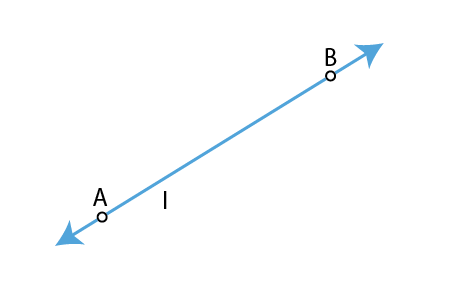To know more about Points, Lines and Planes, visit here.

### Tools of Construction

Tools used for construction:

• Ruler: An instrument used to draw line segments and measure their lengths.
• Compass: Instrument having a pointer on one end and a pencil on the other end. It is used to mark equal lengths and to draw circles and arcs.
• Divider: Instrument having a pair of pointers. It is used to compare lengths.
• Set- Squares: Two triangular pieces – One of them has 45°, 45°, 90° and the other has 30°, 60°, 90° angles at the vertices. It is used to draw parallel and perpendicular lines.
• Protractor: A semicircular instrument graduated into 180° parts. It is used to draw and measure angles.### Line Segment

Line Segment: Part of a line that is bounded by two distinct endpoints.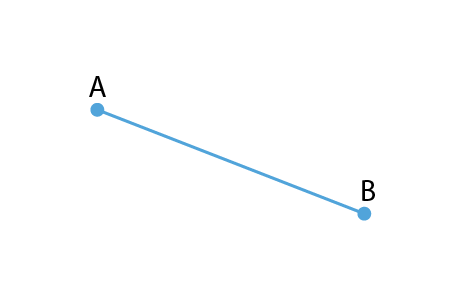To know more about Line Segment, visit here.

### Constructing a Line Segment for a given Length

Steps for constructing a line segment of a given length ‘a’:

(i) Draw a line l and mark a point A on it.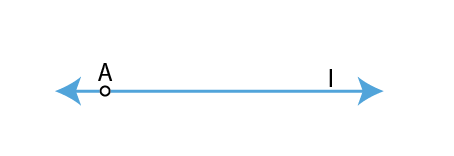(ii) Place the compass on the initial point of the ruler. Open it to place pencil point up to the ‘a’ mark.
(iii) Place the pointer on A and draw an arc to cut l at B. AB is the required line segment.To know different methods to draw a Line Segment, visit here.

### Constructing Copy of a Line Segment

Steps for constructing a copy of a given line segment using ruler and compass together:

(i) Given AB whose length is unknown.

(ii) Fix compass’ pointer on A and pencil end on B. The opening of the instrument now gives the length of AB.

(iii) Draw any line l.

(iv) Placing the pointer on C, draw an arc that cuts l at a point say D. Then, CD = AB.
To know more about Constructing a Copy of a Line Segment, visit here.

### Perpendiculars and Parallels

A line MN meeting another line AB at the right angle is said to be the perpendicular to the line AB.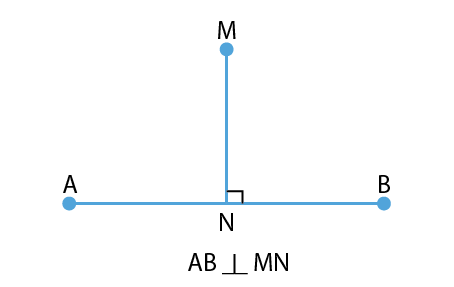If two lines are non-intersecting and are always the same distance apart, then they are said to be parallel lines.
As shown in the figure, AB || CD.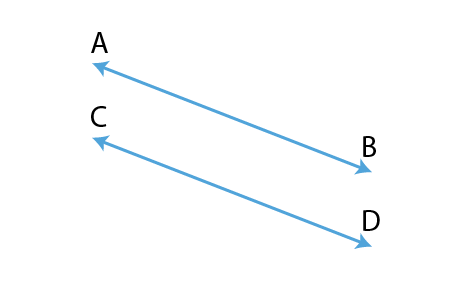### Constructing a Perpendiculars Using a Compass and Ruler

Steps for constructing perpendiculars using compass and rulers:
(i) Given a line I and a point P not on it.
(ii) With P as the centre, draw an arc which intersects line I at two points A and B.
(iii) Using the same radius and with A and B as centres, construct two arcs that intersect at a point, say Q, on the other side.
(iv) Join PQ. Thus,

$$\begin{array}{l}\bar{PQ}\end{array}$$
is the perpendicular to l.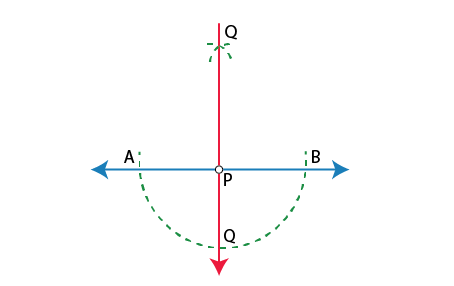### Constructing Perpendicular to a Line through a Point on the Line

Steps to construct a perpendicular to a line through a point on the line:

(i) Place a ruler along a given line l such that one of its edges is along l.

(ii) Place a set square with one of its edges along the already aligned edge of the ruler.

(iii) Slide the set square such that its right-angled corner coincides with the Point P.

(iv) Draw PQ and PQ are perpendicular to l.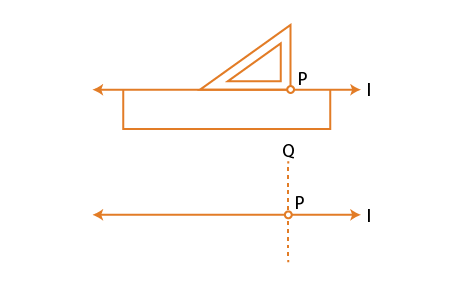To know more about Construction of Perpendicular Lines, visit here.

### Paper Folding Construction

Paper folding method to make perpendiculars:

(i) Let l be the given line and P be a point outside l.

(ii) Place a set-square on l such that one arm of its right angle aligns along l.

(iii) Place a ruler along the edge opposite to the right angle of the set-square.

(iv) Slide the set-square along the ruler till the point P touches the other arm of the set-square.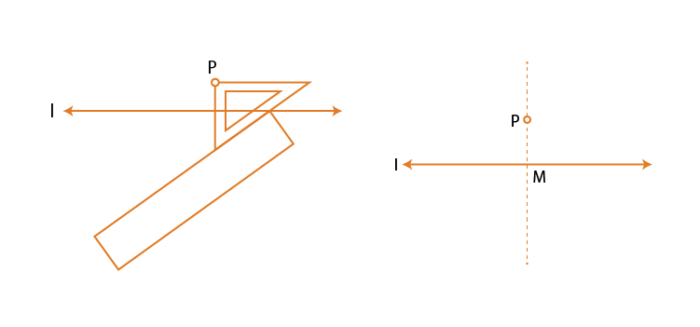### Circle

A circle is a set of all points in a plane that are equidistant from a point i.e. centre of the circle.

To know more about Circles, visit here.

### Construction of a Circle for a given Radius

Steps for constructing a circle using a compass:
(i) Open compass for the required radius.
(ii) Place pointer of the compass on O.
(iii) Rotate the compass slowly to draw the circle.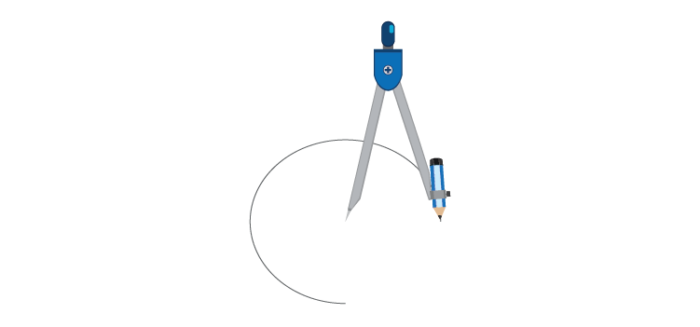To know more about Construction of a Circle, visit here.

## How to Construct an Angle

### Angle Bisector and Its Construction

Steps to construct angle bisectors of a given angle:

(i) With O as the centre, draw an arc that cuts both rays at A and B.

(ii) With B as the centre, draw an arc whose radius is more than half of the length of AB.

(iii) With A as the centre, with the same radius, cut an arc in the interior of ∠BOA

(iv) Mark point of intersection as C. Then, OC is the angle bisector.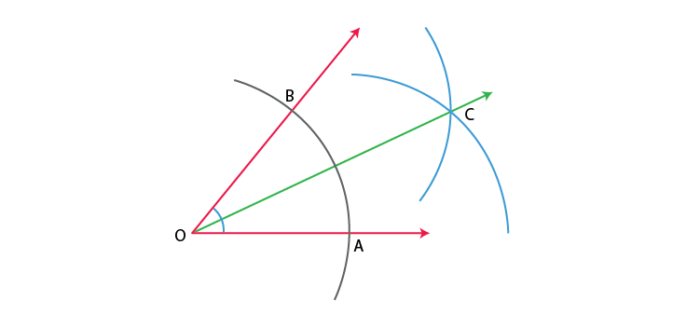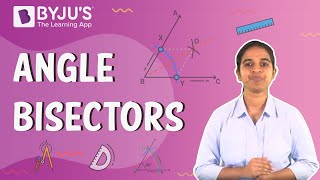### Construction of 30°, 60°, 90°and 120°Angles

(i) Construction of 60° angle: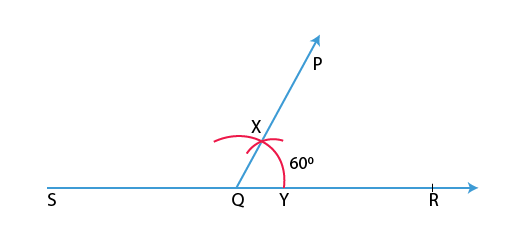(ii) Construction of 120°angle: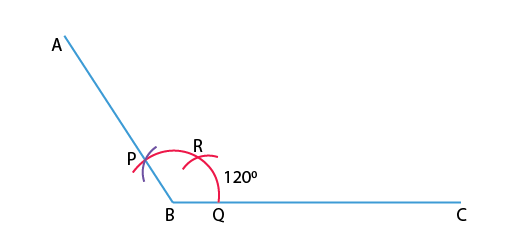(iii) Construction of 90° angle: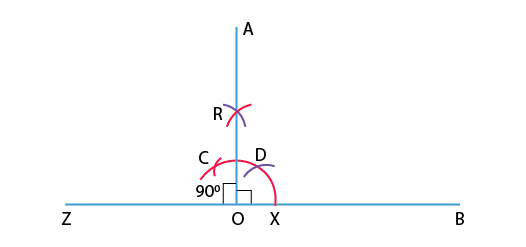(iv) Construction of 30° angle: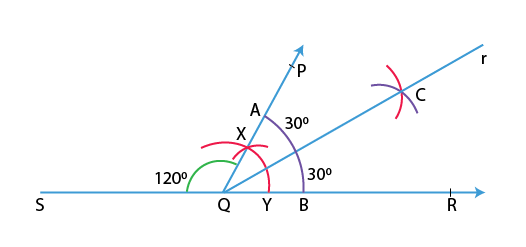To know more about Construction of Angles, visit here.

### Constructing of an Angle with Unknown Measurement

Steps for constructing a copy of an angle with unknown measurement:

(i) Draw a line l and choose a point P on it.

(ii) Place compass’ pointer at A and draw an arc to cut the rays of ∠A at B and C.

(iii) Draw an arc with P as the centre, cutting /at Q.

(v) Place the compasses pointer at Q and draw an arc to cut the arc drawn earlier in R.

(vi) Join PR. This gives ∠P=∠A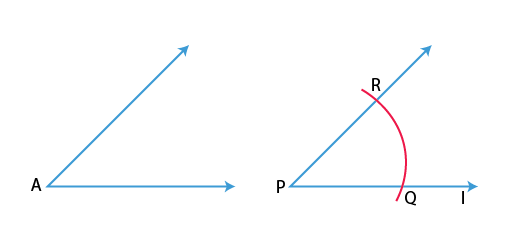### Angles

Angles: Formed by two rays sharing a common endpoint.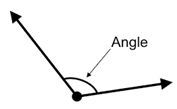To know more about Angles, visit here.

## Frequently asked Questions on CBSE Class 6 Maths Notes Chapter 14: Practical Geometry

### What are some of the uses of practical geometry?

1. Computer graphics 2. Construction of buildings 3. Art 4. Architecture and interior designing 5. Study of orbits and planetary motions

### What is a ‘Line segment’?

In geometry, a line segment is a part of a line that is bounded by two distinct end points and contains every point on the line that is between its endpoints.

### How can a circle be defined?

A circle consists of a closed curved line around a central point. Every point on the line is the same distance from the central point.